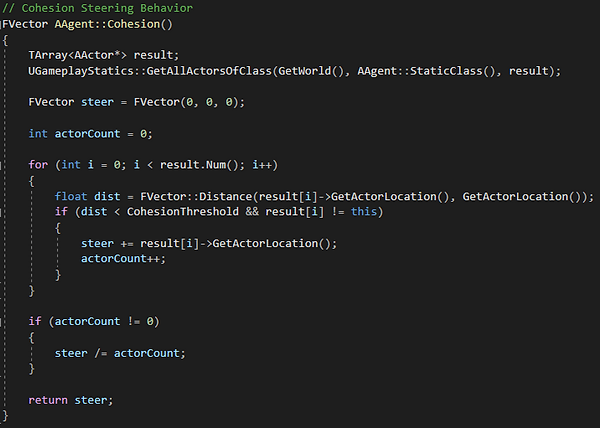top of page##### Steering Behaviors Used
###### Seek

For Seek, I used a simple algorithm to find the direction of the location to seek. To get a better result, I set the z to 0 so that the seek only only done on the X-Y plane. I also wanted to clamp the speed to the max acceleration the agent could go so I divided the distance value by the maximum acceleration so it always returns a value between 0 and 1###### Separate

To get the agents to not touch each other, I decided to use the separation steering behavior. To do this, I found all of the agents that were within a given radius to the current agent. From there I applied a force to repel the agents away from each other. This force is relative to how close the target is to the given radius. To do this, I first subtracted the maximum distance by the distance between the two actors and then I divided it all by the maximum distance. Why I do this subtraction is that I wanted to reverse the effect so that the closer an agent gets to another agent, the more powerful of a resistance force would be added###### Avoidance

Another steering behavior I used to get the agents to actually avoid the obstacles that are put in front of them. To achieve this, the first I needed to do is for the agent to determine if it needs to move to avoid an obstacle. To do this, I did a half done detection, which involved me doing a dot frontward product and then finding if that dot product was within the half cone's threshold, which was found by taking the cosine of the threshold. After it is determined that there is an obstacle inside of the cone, I then take the rightward dot product to determine how far I need to move the agent. From here, I just multiply this dot product with the actor's relative rightward vector and then use the same technique I used in separation to add more force to the agent if it is closer to an object.
Originally, I used multiple raycasts to find where to move the agent. However, I found that this implementation would make the steering a lot more twitchy since it would immediately stop steering after it went out of reach to the raycast, which could even happen if there was a corner of a wall that the two raycasts could not reach.###### Cohesion

To get the agents to actually stay with each other, I used the cohesion steering behavior. For each agent in the scene, I would find all of the agents within a radius (which could be defined by the user). I would then average out all of the agent's positions to get one average location, which the agent would then seek towards. If there are no agents within the given radius, cohesion will then have a direction and strength of 0.###### Align

Finally, to ensure that all of the agents point in the general direction of the target, I used the align steering behavior. How this steering behavior works is that I find all of the agents in a given radius (which once again is determined by the user) and it gets all of the normalized velocities of these agents. After it does that, it finds an average velocity between all of these agents and then it multiplies it by the max speed and then the final result is the directional vector between this velocity and the agent's velocity. Much like cohesion, alignment returns 0 if there are no agents within the given radiusbottom of page